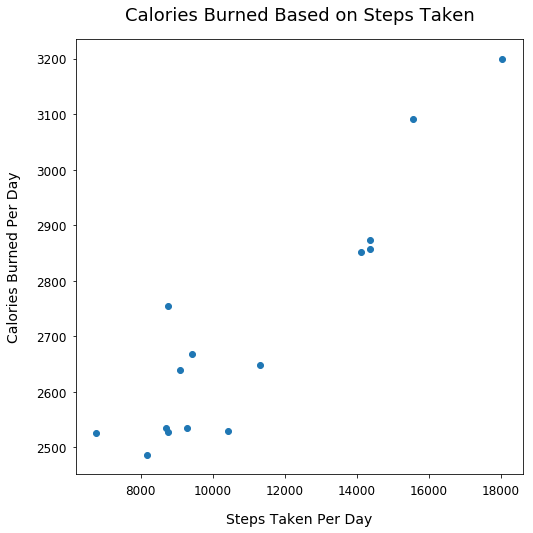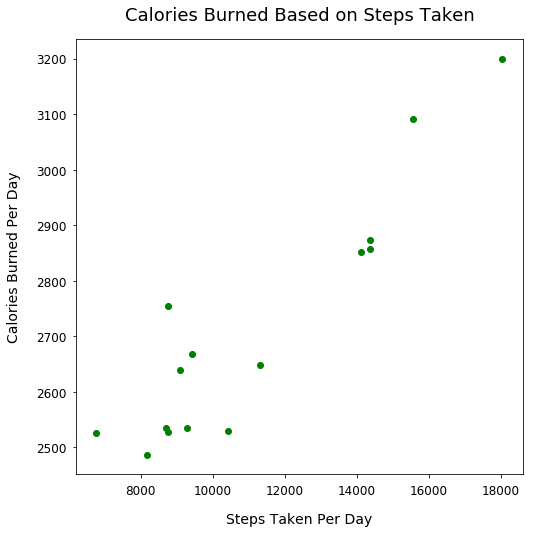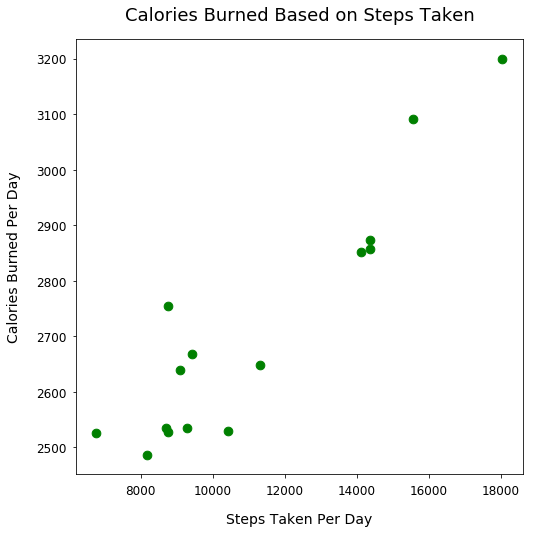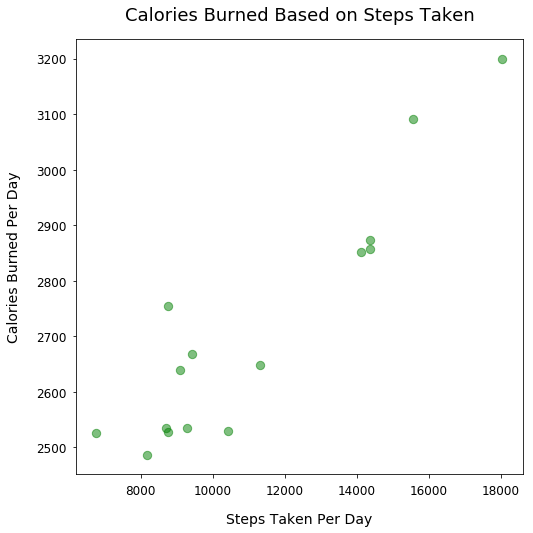Data Visualizations Matplotlib Plotting Tutorial

# Customize Scatter Plot Styles using Matplotlib

This post will cover some basic concepts for styling scatter plots in Matplotlib such as how to adjust:

• Color of scatter points
• Size of scatter points
• Transparency of scatter points

#### Import Modules¶

In :
import matplotlib.pyplot as plt
% matplotlib inline


#### Fitbit Activity Data¶

Below is my Fitbit data of daily step count and daily calories burned over a 15-day period.

In :
calories_burned = [3092, 2754, 2852, 2527, 3199, 2640, 2874, 2649,
2525, 2858, 2530, 2535, 2487, 2534, 2668]

In :
steps = [15578, 8769, 14133, 8757, 18045, 9087, 14367, 11326, 6776,
14359, 10428, 9296, 8177, 8705, 9426]


#### Initial Plot with Generic Plot Style Customizations¶

These initial styles could be applied to any type of plot - whether that be a scatter plot, line plot or a histogram.

In :
plt.figure(figsize=(8, 8))
plt.scatter(steps, calories_burned)
plt.title("Calories Burned Based on Steps Taken", fontsize=18, y=1.03)
plt.xlabel("Steps Taken Per Day", fontsize=14, labelpad=15)
plt.ylabel("Calories Burned Per Day", fontsize=14, labelpad=15)#### Adjust Color of Scatter Points¶

Utilize the c argument for the scatter method and set it to green to make the scatter points green.

You can see additional colors on this Matplotlib documentation page.

In :
plt.figure(figsize=(8, 8))
plt.scatter(steps, calories_burned, c='green')
plt.title("Calories Burned Based on Steps Taken", fontsize=18, y=1.03)
plt.xlabel("Steps Taken Per Day", fontsize=14, labelpad=15)
plt.ylabel("Calories Burned Per Day", fontsize=14, labelpad=15)#### Adjust the Size of Scatter Points¶

Utilize the s argument in our scatter method and pass in the value 75 to make larger scatter points that are easier to see.

In :
plt.figure(figsize=(8, 8))
plt.scatter(steps, calories_burned, c='green', s=75)
plt.title("Calories Burned Based on Steps Taken", fontsize=18, y=1.03)
plt.xlabel("Steps Taken Per Day", fontsize=14, labelpad=15)
plt.ylabel("Calories Burned Per Day", fontsize=14, labelpad=15)#### Adjust the Transparency of Scatter Points¶

Utilize the alpha argument in our scatter method and pass in a numeric value between 0 and 1.

A value of 0 will make the plots fully transparent and unable to view on a white background. A value of 1 is the default with non-transparent points.

We'll make the scatter points slightly transparent so you more easily see the overlap of points near the bottom left of our plot.

In :
plt.figure(figsize=(8, 8))
plt.scatter(steps, calories_burned, c='green', s=70, alpha=0.5)
plt.title("Calories Burned Based on Steps Taken", fontsize=18, y=1.03)
plt.xlabel("Steps Taken Per Day", fontsize=14, labelpad=15)
plt.ylabel("Calories Burned Per Day", fontsize=14, labelpad=15)